# C++ 虚函数的内部实现

## 1. 知识储备

• 什么是继承?

• 什么是虚函数？

在C++中，在基类的成员函数声明前加上关键字 `virtual` 即可让该函数成为 虚函数，派生类中对此函数的不同实现都会继承这一修饰符。

• 为什么需要虚函数？

## 2. C++中类的memory Layout

##### 1. 我们从最普通的一个类说起~
``````class X {
int	x;
float	xx;
static int  count;
public:
X() {}
~X() {}
void printInt() {}
void printFloat() {}
static void printCount() {}
};
``````

 ``` class X { int x; float xx; static int count; public: X() {} ~X() {} void printInt() {} void printFloat() {} static void printCount() {} }; ```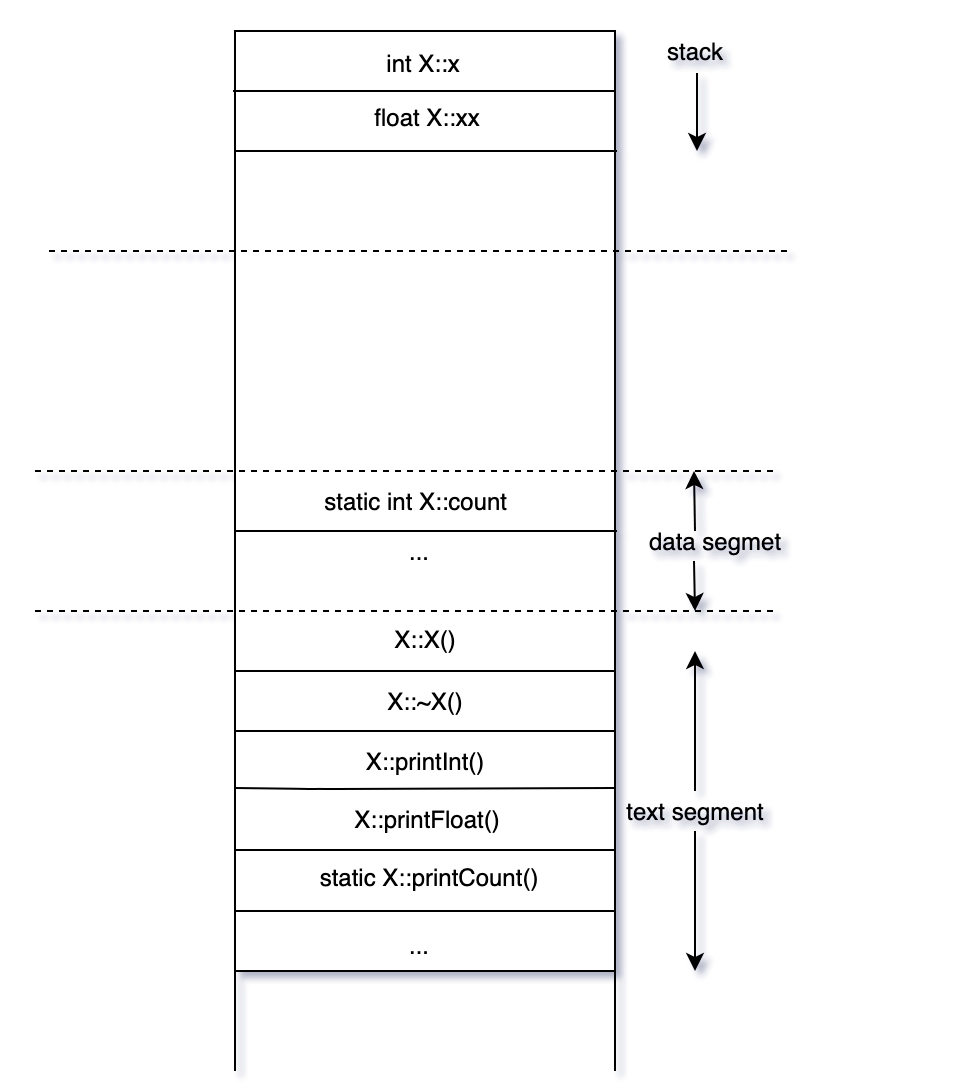##### 2. 含有虚函数的基类的内存分布

`vtable`中的第一个entry包含了当前类及其基类的相关信息，其余entry是函数指针。

 ```class X { int x; float xx; static int count; public: X() {} virtual ~X() {} virtual void printAll() {} void printInt() {} void printFloat() {} static void printCount() {} }; ```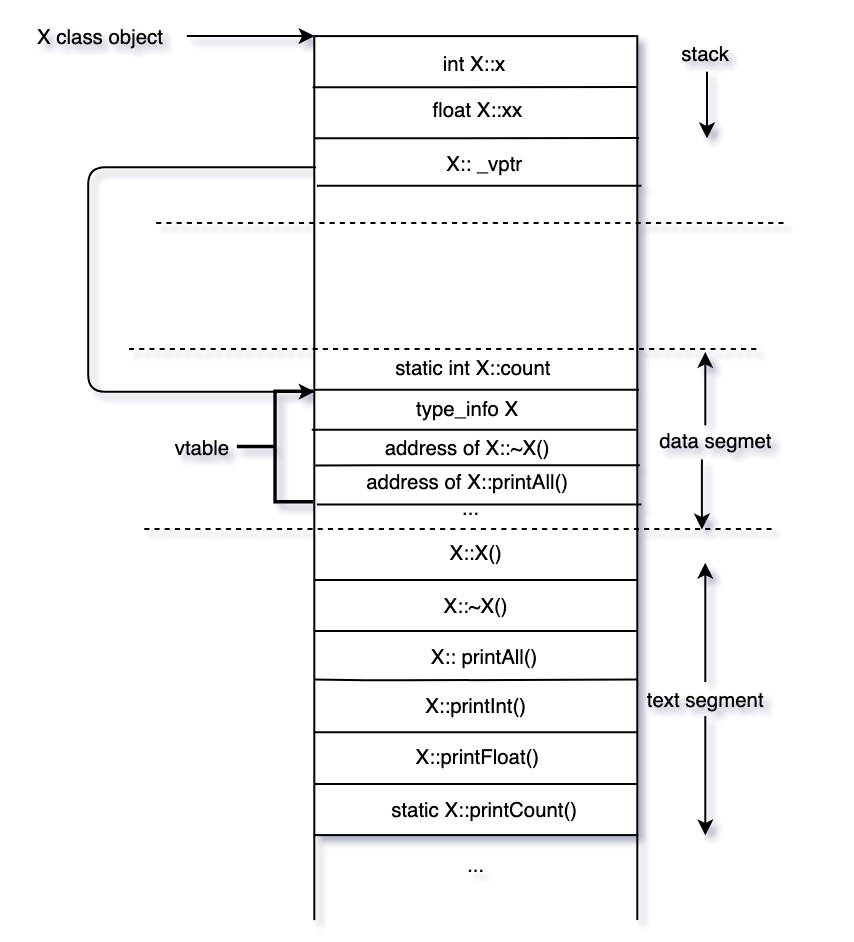##### 3. 含有虚函数的子类的内存分布

 ```class X { int x; public: X() {} virtual ~X() {} virtual void printAll() {} };``` ```class Y : public X { int y; public: Y() {} ~Y() {} void printAll() {} }; ```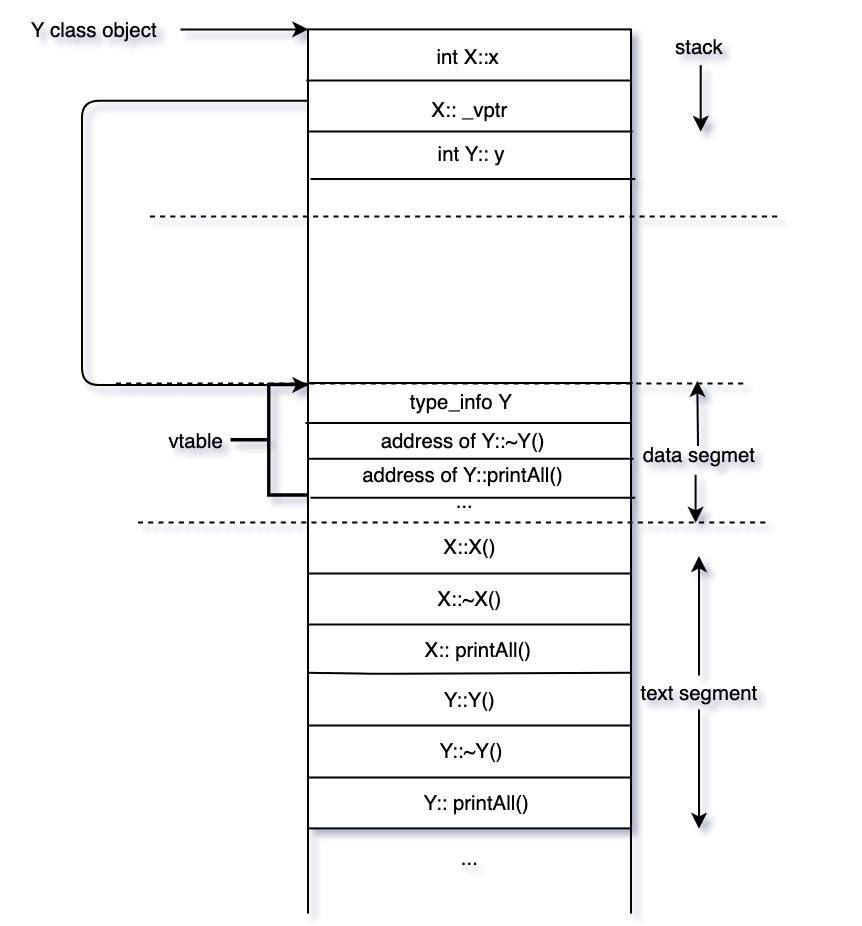##### 4. 含有虚函数、有多继承的子类的内存分布

 ```class X { public: int x; virtual ~X() {} virtual void printX() {} }; ``` ```class Y { public: int y; virtual ~Y() {} virtual void printY() {} };``` ```class Z : public X, public Y { public: int z; ~Z() {} void printX() {} void printY() {} void printZ() {} }; ```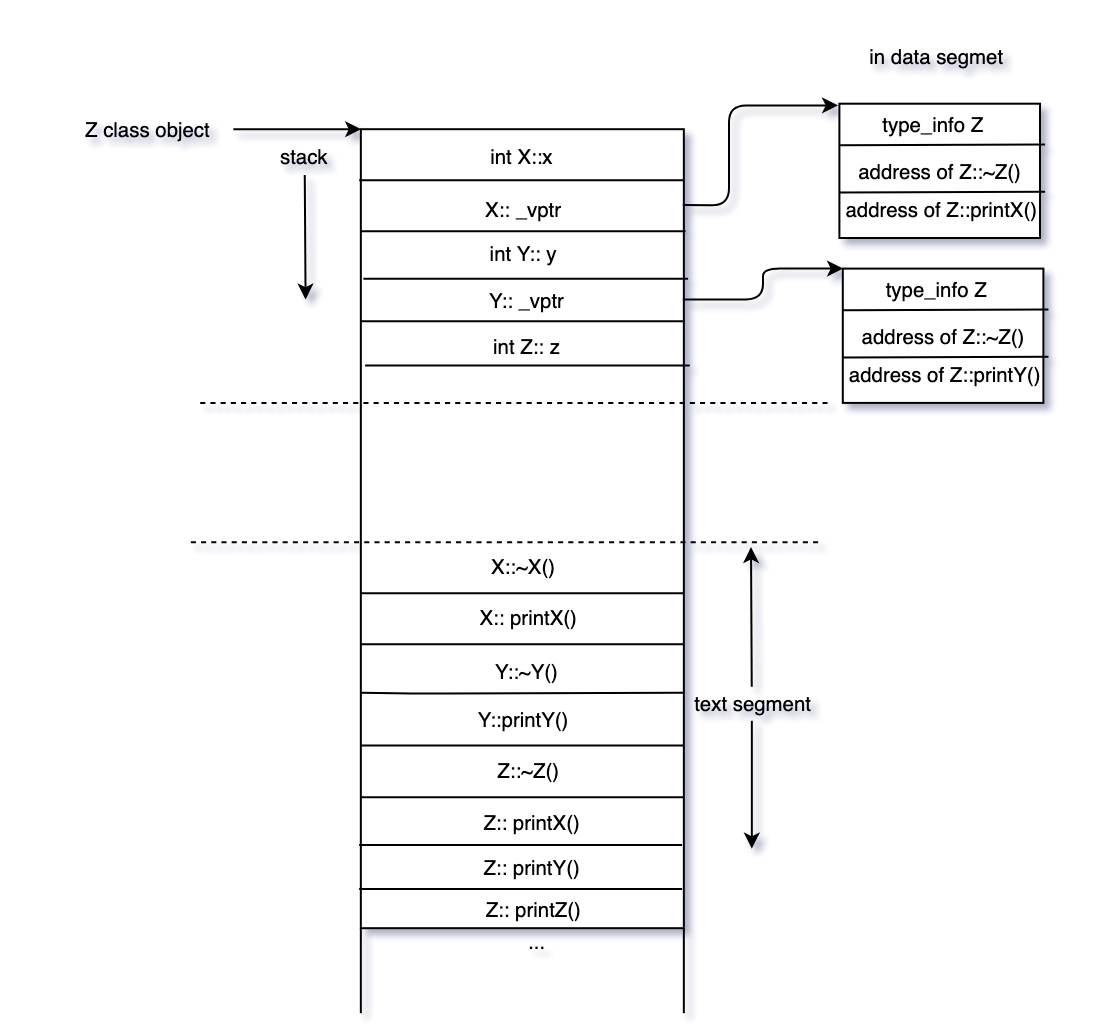## 3. 虚函数的内部实现

``````class X {
public:
int     x;
virtual ~X() {}
virtual void printX() { cout<<"printX() in X"<<endl; }
};

class Y {
public:
int     y;
virtual ~Y() {}
virtual void printY() { cout<<"printY() in Y"<<endl; }
};

class Z : public X, public Y {
public:
int     z;
~Z() {}
void printX() { cout<<"printX() in Z"<<endl; }
void printY() { cout<<"printY() in Z"<<endl; }
void printZ() { cout<<"printZ() in Z"<<endl; }
};

int main(){
Y *y_ptr = new Z();
y_ptr->printY(); // OK
y_ptr->printZ(); // Not OK, Y类的虚函数表中没有printZ()函数

y_ptr->y = 3; // OK
y_ptr->z = 3;// not OK, Y类的空间中没有变量z
}
``````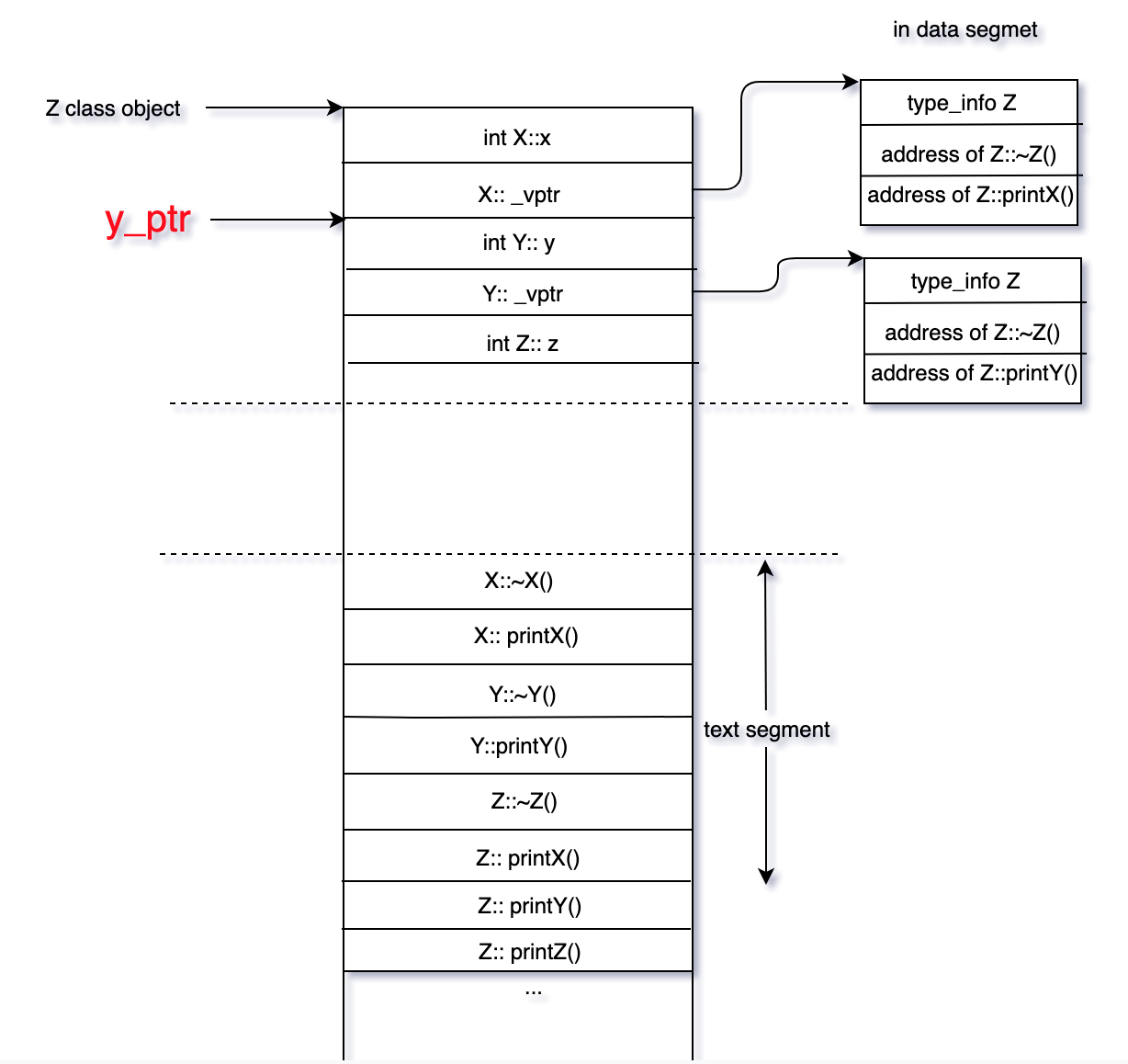`y_ptr->printY()`这行代码，其实会被编译器翻译成如下伪代码

``````((y_ptr->_vptr)->_vtbl)();
``````

## 4. 用几个问题加深理解

#### Q1. 如果将Z类对象赋值给Y类变量，动态绑定还会发生吗？

``````Z zz;
Y yy = zz;
yy.printY();
``````

#### Q2. 如果在基类中不声明某个函数是虚函数，在子类中重写了这个函数，动态绑定还会发生吗？

``````class X {
public:
int     x;
virtual ~X() {}
void printX() { cout<<"printX() in X"<<endl; }
};
class Z : public X {
public:
int     z;
~Z() {}
void printX() { cout<<"printX() in Z"<<endl; }
void printZ() { cout<<"printZ() in Z"<<endl; }
};

int main(){
X *x_ptr = new Z();
x_ptr->printX(); // OK
}
``````

## 5. 总结

1. 一般而言，虚函数表是属于一个类的(one vtable per class)， 位于静态数据区，而虚函数表指针`_vptr`是属于一个类的对象的(one vptr per object).
2. 一个由多继承关系的类会有多个虚函数指针。
3. 虚函数指针的赋值操作是在构造类对象的过程中发生的，之后的赋值操作不会改变`vptr`的值
4. C++标准没有定义动态绑定的具体实现方式，只是陈述了动态绑定的行为。具体的实现与编译器相关。

//www.vishalchovatiya.com/memory-layout-of-cpp-object/

//www.vishalchovatiya.com/part-1-all-about-virtual-keyword-in-cpp-how-virtual-function-works-internally/

//www.learncpp.com/cpp-tutorial/the-virtual-table/

//www.cnblogs.com/yinheyi/p/10525543.html

Tags: